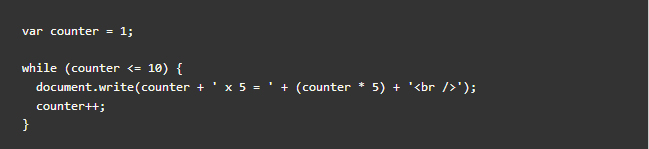#### While Loop

There will be situations where you need to execute a set of code over and over again in programming. It is not practical to write same lines of code again and again. In such situations, you would need to write loop statements to reduce the number of lines.

Loops checks a condition. If it returns true, a code block will run. Then the condition is chacked again and if it still returns true, the code will run again. It repeats until the loop condition returns false.

The most basic form of loops is While Loop. While loop will continue to run as long as condition is true. While loops are generally useful if you do not the exact number of times the loop should run. Here's an example:This while loop writes out multiplication table of 5. Each time the loop is run, each step is written to the page. This loop will continue to run for as long as the condition in the parentheses is true (counter is less than or equal to 10). The second statement inside while loop 'counter++;' increments the counter variable by one.

In this example, the condition specifies that the code should run ten times. A more typical use of a while loop would be when you do not know how many times you want the code to run. It should continue to run as long as a condition is met.

NEXT LESSON